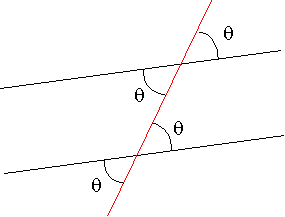Scalars and Vectors

• Scalar: A quantity like mass or temperature which only has a magnitude
• Vector: A quantity like heat flux or force which has both a magnitude and a direction (denoted by a bold faced character, an underlined character, or a character with a arrow on it)
• Vector Addition: Vector Addition follows the parallelogram law described be the figure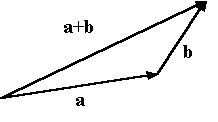• Resolution of a Vector: A vector can be resolved along different directions using the parallelogram rule. The figure shows how one resolves vector c into components a and b which are along the given directions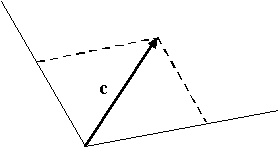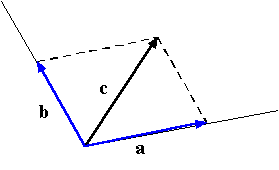• The math you need:
• For a right triangle:

a2+ b2 = c2

tan(q) = b/a

sin(q) = b/c

cos(q) = a/c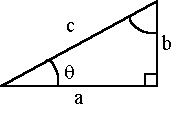• For a general triangle: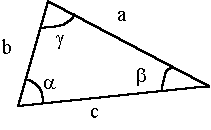a+b+g=180o

Sine law: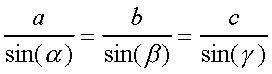Cosine law: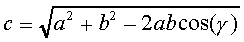o        A line intersecting parallel lines: